Xu, Z., Ni, S., Yu, M., and Huang, L. (2018). "An image-based laser triangulation width model applied in sawn lumber’s external face measurement," BioRes. 13(4), 7371-7380.

#### Abstract

The yield of sawn lumber has been largely governed by the identification accuracy of the external face width. However, the traditional method cannot measure the external face width with high accuracy and low error. In view of this, an image-based laser-triangulation width measurement model was established to measure the width of sawn lumber’s external face in an effort to overcome the deficiency of the traditional method. A hypothesis was established from the theoretical model, as follows: when the laser line is cast on the external face and the reflected light is captured by the camera with a certain angle, the width resolution will be the least affected and will even depend on the resolution of the camera under precise conditions. Data from contrasting experiments suggested that a particular angle has a width-detection error 0.15% and accuracy 0.177 mm, which absolutely satisfies the need of wood industry detection online. Furthermore, this paper gives a theoretical proof of conjecture from the perspective of optical principles and mathematical analysis.

An Image-based Laser Triangulation Width Model Applied in Sawn Lumber’s External Face Measurement

Zhaojun Xu,a,* Shenjian Ni,a Maolin Yu,b and Lei Huang b

The yield of sawn lumber has been largely governed by the identification accuracy of the external face width. However, the traditional method cannot measure the external face width with high accuracy and low error. In view of this, an image-based laser-triangulation width measurement model was established to measure the width of sawn lumber’s external face in an effort to overcome the deficiency of the traditional method. A hypothesis was established from the theoretical model, as follows: when the laser line is cast on the external face and the reflected light is captured by the camera with a certain angle, the width resolution will be the least affected and will even depend on the resolution of the camera under precise conditions. Data from contrasting experiments suggested that a particular angle has a width-detection error 0.15% and accuracy 0.177 mm, which absolutely satisfies the need of wood industry detection online. Furthermore, this paper gives a theoretical proof of conjecture from the perspective of optical principles and mathematical analysis.

Keywords: Laser triangulation; Width detection; Sawn lumber; External face

Contact information: a: College of Materials Science and Engineering, Nanjing Forestry University, Nanjing, 210037, China; b: College of Mechanical and Electronic Engineering, Nanjing Forestry University, Nanjing 210037, China; * Corresponding author: 105385291@qq.com

INTRODUCTION

The manufacturing of wood starts with cutting the logs into sawn lumber with a given thickness, resulting in two sides with wanes and two outer surfaces (Funck et al. 2003). These surfaces can be named the internal face (near to the pith) and the external face (far from the pith) in terms of their distances to the log pith (Fig. 1). Manual measurement, machine vision, and laser scanning are methods usually applied in the external face identification. However, these methods cannot accurately measure the width of the external face. The manual one demands experienced workers and low efficiency since it is unable to measure online. The second one identifies the external surface by extracting the color characteristics, which is efficient but easily leads to misjudgement because the color difference between the wane and other defects, especially in image segmentation (Funck et al. 2003). Laser scanning, notwithstanding, makes up the shortcomings of the first two, yet the width measurement accuracy is low. The chroma scanning series of LMI3D, for example, has 0.2 mm accuracy in height and 8 mm accuracy in width.

Laser triangulation measurement technology has been applied to improve the production in the industry by virtue of high accuracy (Tao and Liu 2010; Lin et al. 2012; Lee et al. 2013). For example, Xiang et al. (2017) built an improved laser triangulation system to measure the melt level. Li et al. (2018) used ployview optics to measure the edge based on triangulation. However, a study concerning image-based width measurement with laser triangulation is needed. This paper looks to address this issue by building an image-based width measurement model according to laser triangulation to accurately detect the sawn lumber’s external face width. The principle of the model is that the laser source generates a beam line focused on the measured surface with a certain angle, and the oblique camera captures the reflection line image. The real thickness value can be precisely obtained after calculating the height of the collected image (Keferstein and Marxer 1998). A continuous profile characterized by one horizontal and two slopes is obtained and the external face can then be identified.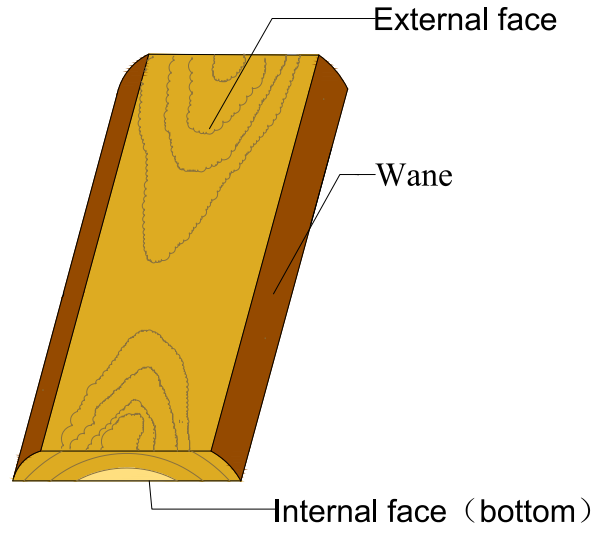Fig. 1. Sawn lumber

EXPERIMENTAL

Detection Model Building

The width model is proposed in terms of geometrical optics independent of the camera effect, lens distortion, phase difference, and other factors of the imaging system. The optical system is based on the paraxial optical conditions, i.e., the imaging process of the camera simplified as pinhole camera imaging (Ray 1994; Forsyth and Ponce 2012).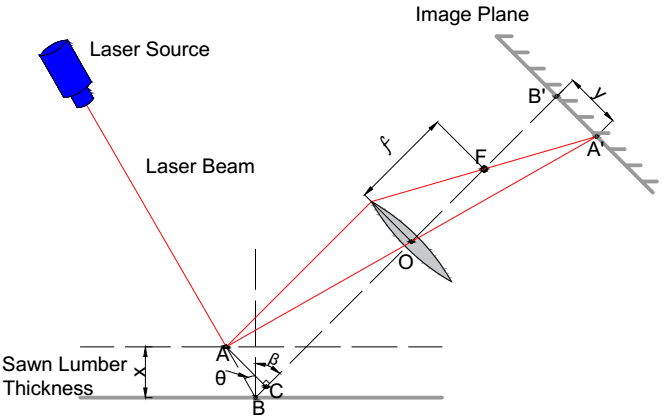Fig. 2. Thickness detection principle

In the thickness model shown in Fig. 2 as seen from the side, the laser source emits a laser beam to the external face of the test sawn lumber. Since the surface of the wood is rough, the reflected light is diffuse, and the reflection law is satisfied. Point is the optical center of the lens of the camera (the intersection of optical axis BB’ and reflection line AOA’) ; point B is the incidence position on the conveyor belt without the test-piece; point B’ is the counterpart of B in the camera imaging plane; and the line segment BB’ coincides with the optical axis; point F is the focus of the lens (the intersection of line BB’ and the other reflection line) and the length of the OF is the focus length. When a nonzero thickness specimen is placed onto the belt, the laser beam will touch the top surface at point A, and A’ is the corresponding point in the imaging plane.

Assume: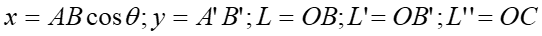where is the real thickness of the test-piece; is the measured height in camera imaging (unit: pixels); is the object distance (test-piece with a zero thickness); L’ is the image distance; and L’’ is the object distance either (test-piece with a nonzero thickness). f is the focal length of the camera lens. θ is the angle between the incident laser beam and the normal of the transfer platform. β is the angle between the optical axis of the camera and the normal.

When the camera is selected and fixed, θ, β, f, and L’ are fixed values. Equation 1 can be obtained as follows: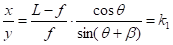(1)

where kis the height converting factor, which is the ratio between and the actual test-piece thickness x. Equation 1 shows that the value of kis determined by the focal length of the lens (f), object distance without test-piece (L), and the angle between the optical axis of the lens and the laser beam (θ and β) together. Once kis determined, the lumber thickness x can be easily calculated from the image.

The width model is shown in Fig. 3, which is a top view of Fig. 2. The object distance is transformed from L to L’’ when the test-piece is placed on the conveyor with a certain thickness.(2)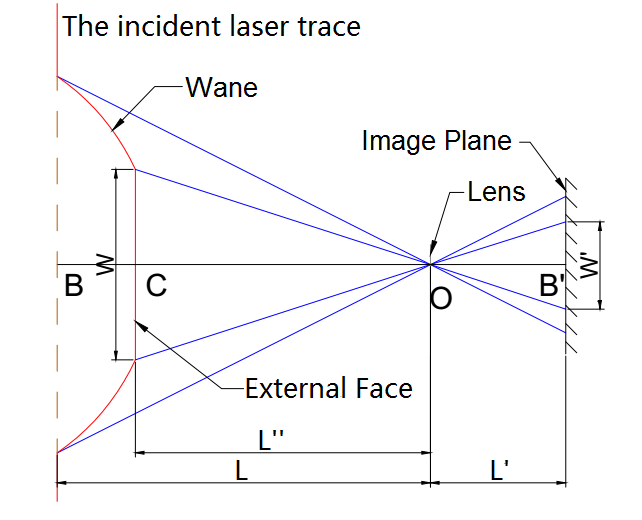Fig. 3. Schematic diagram of width measurement

Several interesting features can be found from Eq. 2:

(a) k2 is independent of W.

(b) When θ+β = 90° and 0°<θ<90°, k2’ will be independent of (the actual thickness of the text-piece) and equal to k(a constant value depending on the camera model).

(c) When θ+β ≠ 90° and 0°<θ<90°, k2’ will have a liner relationship with x, which means that k2’ is affected by the thickness.

The above-mentioned features are of great importance, because the effect of the sawn lumber thickness is considered when the image pixel is used to calculate the actual width due to the oblique of the laser beam. With these characteristics, the influence of different gauge width on the calibration experiment has can be ignored. Once k2 is determined, the timber external width W can be easily calculated from the image.

Device and Materials

The experimental devices were composed of a camera, a laser light source, a mechanical frame, a control device, and a computer are shown in Fig. 4. The laser light source comes from ProPhotonix company (Salem, NH, USA) (catalog number: D-660-010-0250-L01-S-90-S-S-2, wavelength: 660 nm, fan angle: 90°, power: 10 mw). The camera comes from Point Grey company (BC, Canada) (model number: GS3-U3-23S6C-C, resolution ratio: 1920*1200, the largest collection frames per second: 162, interface: USB3). The lens comes from Nikon company (Shanghai, China) (model number: ML-U1614MP9), focal length: 24mm.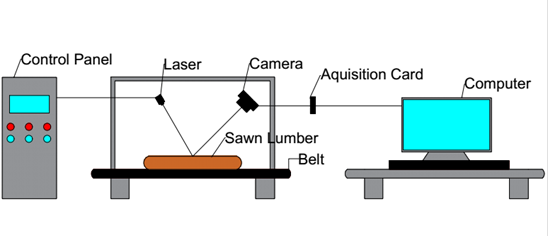Fig. 4. Diagram of detection device

Nine pieces of Chinese fir (Cunninghamia lanceolata (Lamb.) Hook.) sawn lumber with distinct edges were used, which had an average width of 180 mm and the average length of 800 mm. The thicknesses of these sawn lumber pieces ranged from 20 mm to 60 mm with a 5 mm increment. The precise thickness value of the sawn lumber was calibrated by gauges, ranging from 5 mm to 25 mm with a 0.5 mm increment and from 30 mm to 60 mm with a 5 mm increment. Meanwhile, eleven pieces of custom-made gauges with a fixed width were used for width calibration. These pieces had thicknesses ranging from 10 mm to 60 mm with a 5 mm increment, and each gauge had 6 marked test lines on their surface.

Method

Based on the model mentioned above, the height converting factor kcurves and width converting factor k2’ were calibrated to verify the model’s correctness. The camera was adjusted to the condition that the clearest image could be captured during the experiment. There were 56 lines marked along the width direction on each test-piece measured as the “real value” by a caliper. The laser beam overlapped these marked lines during the experiments, obtaining a series of different width value treated as the “measured value”. The error between the “real value” and “measured value” was utilized to evaluate the accuracy and reliability of the detection system. The camera with the inclination angle of the optical axis (β) was fixed as 45° in all experiments. The angle between the laser beam and the normal of the conveyor plane was set to three different angles (θ), i.e., 30, 45, and 60°, during the experiments.

Process

In the first step, the calibration in thickness direction was implemented via appropriately placing a series of standard thickness gauge blocks in the detection system to obtain the linear regression analysis with the “measured” and standard thickness value. The width calibration was carried through by properly placing a batch of custom-made gauge blocks to collect a series of width pixel value. The actual width value was divided by the width pixel value to obtain the width-converting factor (k2), which was analyzed by linear regression with the actual height of these gauge blocks. The verification test had a total of 246 sets of data.

In the second step, the verification tests were carried out with the laser beam of incline angles at 30, 45, and 60 degrees. Each piece of the sawn lumber was marked with 56 lines on its external face, and the lengths of these lines were measured by both a caliper and a camera, which were respectively treated as the “real value” Ln0(i) (n representing the No. of the marked lines; i representing the No. of the sawn lumber) and the “measured value” Ln(i). A total of 1512 sets of data were obtained in the verification tests.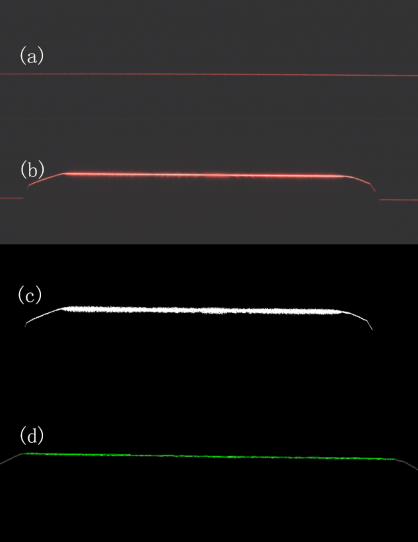Fig. 5. Image processing. Line (a) datum plane image; line (b) original detection image; line (c) binary image; line (d) final processing image

In the third step, the laser stripe center was extracted from an image, which is one of the most important measurement procedures when using structured light techniques (Sun et al. 2015). As shown in Fig. 5, line (a) represented the image without the test-piece, which was horizontally treated as the reference line; line (b) was the image with the sawn lumber; line (c) was the binarization image, where the entire contour line of the sawn timber section and the intense scattering of the beam caused by the rough surface can be seen, by OTSU algorithm, a method applying to automatically perform image thresholding in the computer vision and the image processing (Sezgin and Sankur 2004; Sha et al. 2016); line (d) was the final image, by extracting the central line from the binary image after removing some absolute slope values larger than value 0.1 segments, which may be caused by the distortion effect of the rough surface of the sawn lumber, processed by Hough transform algorithm (Duda and Hart 1972; Mochizuki et al. 2009). Then the height of a test-piece and the width of the external face could be calculated using the model.

RESULTS AND DISCUSSION

Calibration and Verification Results

The calibration regression curves in the thickness direction were plotted in Fig. 6. The correlation coefficients were all above 0.999, which verifies the laser triangulation technology high linearity in the thickness direction.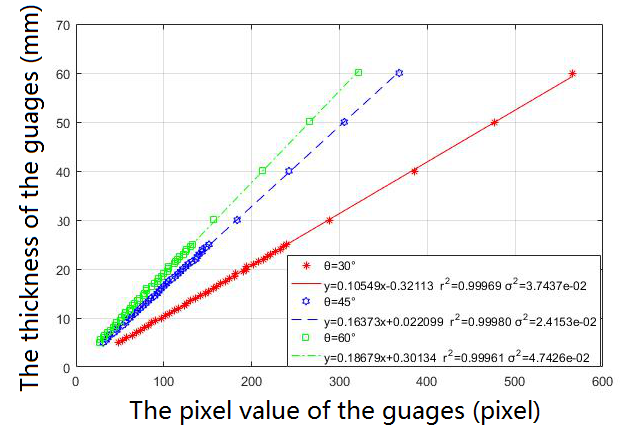Fig. 6. Fitting curves and correlation coefficient of test-pieces height

The width direction calibrations are shown as in Fig. 7. According to Eq. 2, when the angle θ equals 30 or 60 degrees, i.e. θ+β equals 75 or 105 degrees, and the width converting factors had a very good linearity with respect to the test-piece thickness; when the angle θ equals 45 degrees, i.e. θ+β equals 90 degrees, the width converting factor was a constant independent of the test-piece thickness, which is noted above.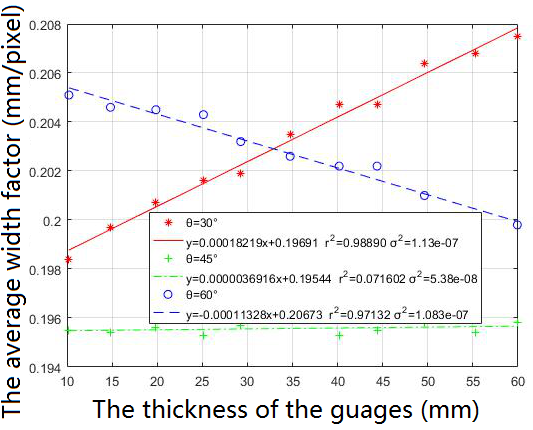Fig. 7. The relationship between the width converting factor and gauges thickness

Error Analysis

Because there were 504 samples in each angle, the experimental error should be subject to the normal distribution. As shown in Fig. 8, it was roughly in line with the normal distribution at three angles, while the relative error of 45 degrees was closer to zero (even close to standard normal distribution), which means the model accuracy at 45 degrees was better than 30.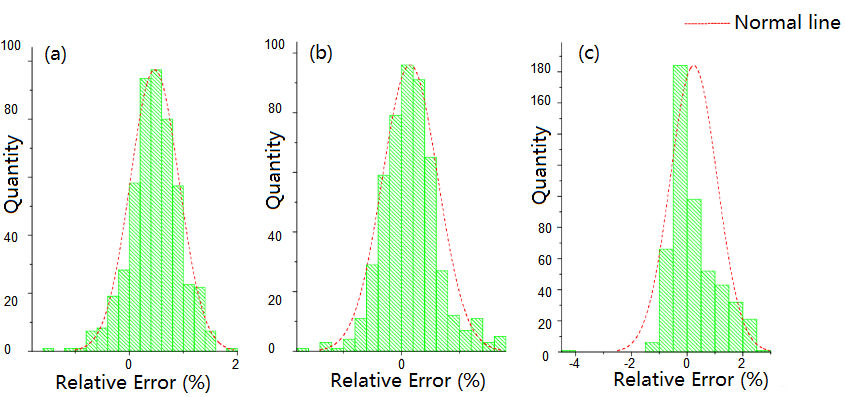（a）θ =30° （b）θ =45° （c）θ =60°

Fig. 8. The distribution of relative detection error under three angles

Table 1 shows the data calculated by Eqs. (3) to (5). It is worth noting that all the average variances (s) of 30 and 45 degrees were close, while at 60 degrees it was much bigger, which would explain the features of Fig. 8. Despite this, the error rate (e) of 30 degrees were significantly higher than for the other angles. Also, the accuracy (a) of 45 degrees was less than 0.18 mm; 45 degrees was judged to be the best angle, as it was the most stable and accurate, such that it can be completely applied into genuine industrial production.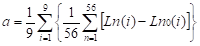(3)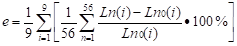(4)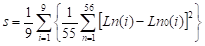(5)

Table 1. The Accuracy, Error Rate, and Stability of the System at Three Angles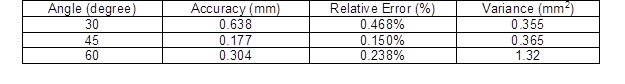To further analyze the effect of the three factors, θβ and x, in Eq. (2), θβ and were used as variables to create a ternary function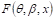for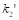: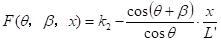(6)

Considering that θβ, and x had some random errors, Taylor’s formula was used to perform a first-order expansion of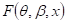ignoring the high order Lagrangian residues: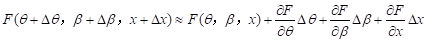(7)

where: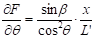(8)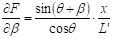(9)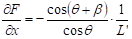(10)

For the microscale,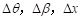had little influence on. Equation 7 contains three factors that influence, among which Eq. 8 represents the influence of θ, Eq. 9 represents the effect incurred by β, and Eq.10 represents the influence of β. When θ and β were both near 45 degrees, the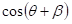value was always near zero, and there were only two factors—Eqs. 8 and 9—that affected. Other angles have three factors, that is to say the accuracy of 45 degrees was best and closer to standard normal distribution. However, 30 degrees and 60 degrees, affected by the combination of Eqs. 8, 9, and 10, so that these circumstances deviated from standard normal distribution

According to the Gaussian imaging principle of geometric optics, the light was parallel to the optical axis (ideal path) in the paraxial region and converges at a point on the image plane through the focal point of the lens, which is called the focal point of the object where the image is the clearest. Inversely, it would leave a blurred light spot in the phase plane resulting in image distortion. Combined with Fig. 9, when the angle of incident light was 45 degrees, the Gaussian imaging rule was satisfied well. Simultaneously, with the angle of 30 degrees and 60 degrees, the reflected light was not parallel to the optical axis, leading to the reflected light propagating away from the ideal path and no longer intersecting with the focus and making the image distort and bias. Therefore, using the image recognition technology for the identification would have errors and then measurement accuracy would decline.

In summary, the influence of some factors that affect width resolution have been analyzed from an optical and mathematical point of view. The results shows when the detection system is set up as laser beam and camera incline angle both 45 degrees, it exhibits the highest accuracy and lowest error rate.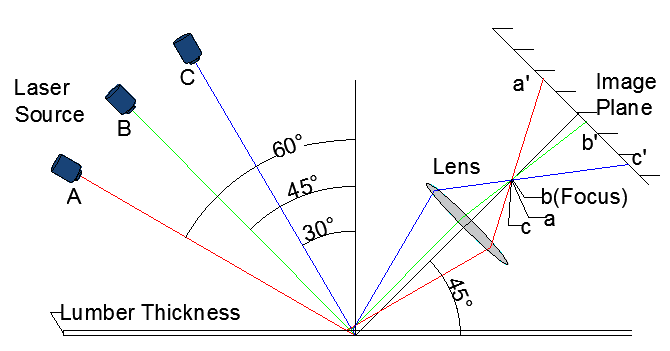Fig. 9. Error analysis optical path

CONCLUSIONS

1. The width and thickness of sawn timber can be accurately measured simultaneously by the model; thus the external surface can be identified through the geometric contour of sawn lumber avoiding the error caused by wood color and improving the width accuracy.
2. The model can accurately measure the width of sawn wood with different thickness.
3. This method can be used not only to measure the width of sawn lumber, but also the cracks, warp, and geometric profile of wood products.

ACKNOWLEDGEMENTS

This work was supported by the Priority Academic Program Development of Jiangsu Higher Education Institutions and the “Thirteenth Five-Year Plan” key development program for financial help, project number: 2016YFD0600703.

REFERENCES CITED

Duda, R. O., and Hart, P. E. (1972). “Use of the Hough transformation to detect lines and curves in pictures,” Cacm 15(1), 11-15. DOI: 10.1145/361237.361242

Forsyth, D. A., and Ponce, J. (2002). Computer Vision: A Modern Approach, Pearson, New York.

Funck, J. W., Zhong, Y., Butler, D. A., Brunner, C. C., Forrer, J. B. (2003). “Image segmentation algorithms applied to wood defect detection,” Computers & Electronics in Agriculture41(1), 157-179. DOI: 10.1016/S0168-1699(03)00049-8

Keferstein, C. P., and Marxer, M. (1998). “Testing bench for laser triangulation sensors,” Sensor Review 18(3), 183-187. DOI: 10.1108/02602289810226408

Lee, K. C., Yang, J. S., and Yu, H. H. (2013). “Development and evaluation of a petal thickness measuring device based on the dual laser triangulation method,” Computers & Electronics in Agriculture 99(9), 85-92. DOI: 10.1016/j.compag.2013.09.001

Lin, L. Z., Cao, C. B., Wang, T., Huang, Z. (2012). “The Q value measurement of the shadow mask surface based on laser triangulation measurement technology,” Advanced Materials Research 452-453, 1465-1469. DOI: 10.4028/www.scientific.net/AMR.452-453.1465

Li, Y., Kästner, M., and Reithmeier, E. (2018). “Triangulation-based edge measurement using polyview optics,” Opt. Laser. Eng 103, 71-76. DOI: 10.1016/j.optlaseng.2017.11.015

Mochizuki, Y., Torii, A., and Imiya, A. (2009). “Point Hough transform for line detection,” J. Vis. Commun. Image R. 20(4), 242-253. DOI: 10.1016/j.jvcir.2009.01.004

Ray, S. F. (1994). Applied Photographic Optics: Lenses and Optical Systems for Photography, Film, Video, and Electronic Imaging, Focal Press, Waltham, MA, USA.

Sezgin, M., and Sankur, B. (2004). “Survey over image thresholding techniques and quantitative performance evaluation,” J. Electron. Imaging 13(1), 146-148. DOI: 10.1117/1.1631315

Sha, C., Hou, J., and Cui, H. (2016). “A robust 2D Otsu’s thresholding method in image segmentation,” Journal of Visual Communication & Image Representation 41, 339-351.DOI:10.1016/j.jvcir.2016.10.013

Sun, Q., Chen, J., and Li, C. (2015). “A robust method to extract a laser stripe centre based on grey level moment,” Opt. Laser. Eng. 67, 122-127. DOI: 10.1016/j.optlaseng.2014.11.007

Tao, H., and Liu, W. (2010). “Measurement system for liquid level based on laser triangulation and angular tracking,” Journal of Computers 5(9), 1444-1447. DOI: 10.4304/jcp.5.9.1444-1447

Xiang, S., Pan, F., Xiang, K., et al. (2017). “Melt level measurement for the CZ crystal growth using an improved laser triangulation system,” Measurement 103, 27-35. DOI: 10.1016/j.measurement.2017.02.018

Article submitted: May 21, 2018; Peer review completed: July 13, 2018; Revised version received: July 18, 2018; Accepted: July 22, 2018; Published: August 14, 2018.

DOI: 10.15376/biores.13.4.7371-7380